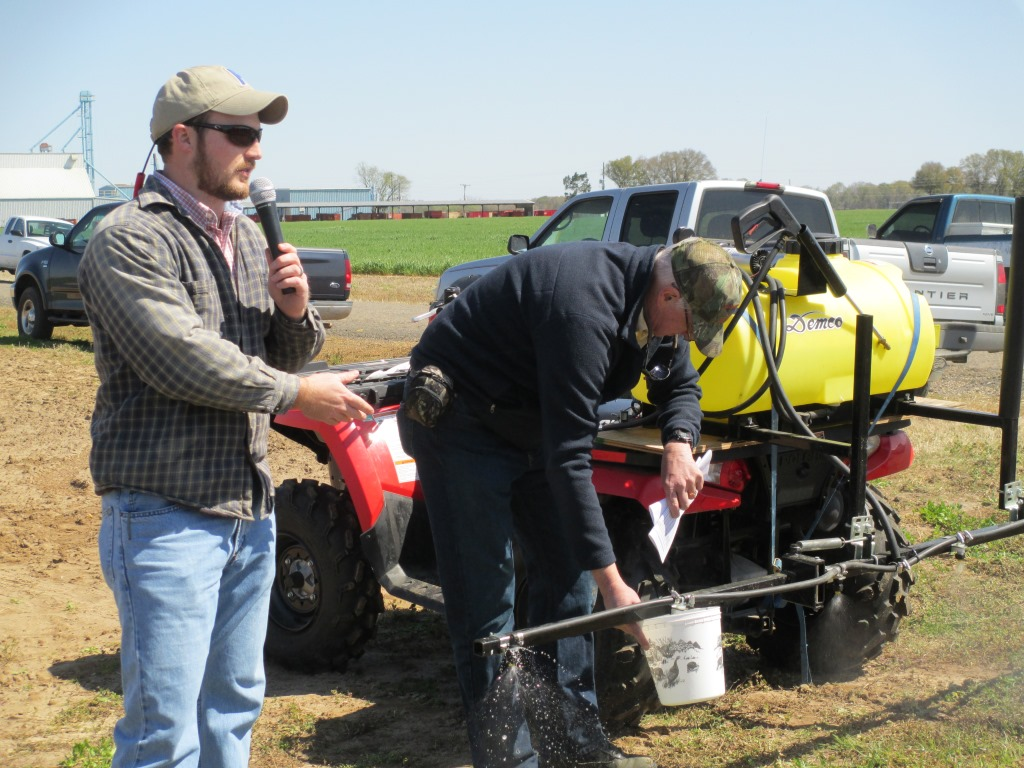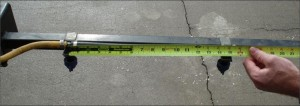# Calibration of Pesticide Applicators: Boom SprayersIt is important to calibrate your pesticide applicators on a regular basis especially as you head into the new crop season.

Now is a good time to calibrate your boom sprayers as you head into the new crop season.  Calibration is not a difficult process if you understand a few principles of calibration.  It is important to calibrate your pesticide applicators on a regular basis to ensure that output from each nozzle is consistent and the desired application rate is achieved.

The following information is from the UF/IFAS publication entitled Calibration of Herbicide Applicators by J. A. Ferrell, B. A. Sellers, and R. Leon:

If a pesticide applicator is not properly calibrated, the pesticide will probably be applied below or above the desired application rate. Under-application of pesticides generally leads to a lack of pest control and poor pesticide performance, while over-application will cost more money than necessary and may also unintentionally result in harm to desirable species.

The two most common methods for sprayer calibration are discussed below.

## The 5940 Method

The 5940 equation is a very accurate way to determine sprayer output (Equation 1). (Each term in this equation is discussed in order, from top and first, to bottom and last.)

Equation 1

GPA = (5940*GPM) / (MPH*W)

GPM, Gallons Per Minute

GPA, Gallons Per Acre

MPH, Miles Per Hour

W, Spray Width of One Nozzle in Inches

5940, Constant

GPM – Gallons Per Minute. The amount of water discharged from a nozzle in 1 minute. This measurement is achieved by turning on the sprayer and holding a measuring cup underneath the nozzle for one minute. Next, transfer the water obtained into a measuring device that will accurately quantify the water in terms of either fluid ounces or milliliters. Next, convert that amount of water from ounces or milliliters to gallons (since gallons-per-minute is the desired unit).

One gallon consists of 128 fluid ounces or 3786 milliliters. To convert to gallons, divide the amount of water acquired in one minute by 128 or 3,786, depending on whether the container you are using measures the water volume in terms of ounces or milliliters. The result of this calculation is gallons per minute (GPM).

For the most accurate measurement, collect water from multiple nozzles to ensure that output is similar across the boom. (It is common for a nozzle to become plugged, and this practice will identify any malfunctioning nozzle). You can also pour the samples together to determine an average GPM.Figure 1. Measuring nozzle spacing to determine “W”
Credit: J. Ferrell – UF/IFAS

W – Spray Swath (or Width). A measurement in inches describing an area covered by one nozzle. If calibrating a boom sprayer, this measurement is the spacing between the nozzles (Figure 1). Common spacing for flat fan nozzles ranges between 15 and 24 inches (see manufacture specifications). If using a boomless nozzle (Figure 2), W will be the width of the pattern for that nozzle. One boomless nozzle will commonly spray a pattern of 12 -15 feet. In that case, W for that nozzle will be between 144 and 180 inches.

MPH – Miles per hour. Most equipment will have a somewhat reliable speedometer. Nonetheless, check the accuracy of the instrument periodically because speed is directly linked to sprayer output in gallons per acre (GPA). If speed is doubled, GPA decreases by half; therefore, small changes in ground speed cause large changes in the amount of pesticide being applied. To check speed, measure off an area (usually 100 feet) and time how long it takes to travel that distance. If using a tractor, make sure that the engine speed (RPM) and transmission gear are at the same settings as when used for spraying. After you have traveled the course, use the following equation:

MPH = (distance (ft)*60) / ( time (sec)*88)

After GPM, W, and MPH have been determined, plug these values into Equation 1 [GPA = (5940*GPM) / (MPH*W)], and solve.

Changing GPA. Equation 1 will allow you to determine sprayer output in terms of gallons-per-acre. However, if GPA is too high or too low, change GPM (by increasing pressure or changing sprayer nozzles) or change the driving speed.

Increasing spray pressure will increase GPM, but only slightly. To illustrate, spray pressure will have to be quadrupled in order to double GPM. Since most spray nozzles are not rated to operate over such a large range of pressures, adjusting pressure is simply a way to “fine tune” sprayer output. Large adjustments in GPM are best achieved by changing to different size nozzles or changing driving speed.

What GPA is common? GPA varies depending on the type of application. As a general rule, carrier volumes that range from 15 – 30 gallons per acre will perform very well. If a specific GPA is desired, Equation 1 can be reorganized – as in the example below – to solve for GPM.

Equation 2

GPM = (GPA*MPH*W) / 5940

In this example, inputting the desired GPA, speed (MPH), and nozzle spacing (W), will give the exact amount of water that must be caught from 1 nozzle in 1 minute (GPM). However, the equation will give the answer in gallons per minute. Since gallons are difficult to measure with accuracy, convert the answer to ounces or milliliters. Multiplying GPM by 128 (for ounces) or 3786 (for milliliters) will give the amount of ounces or milliliters that will need to be caught from 1 nozzle in 1 minute to achieve the desired GPA.

## The 1/128th Acre Method

The 1/128th acre method is a simplified form of calibration based on spraying 1/128th acre. There are 128 ounces per gallon; therefore, the number of ounces sprayed per 1/128th acre is equal to the number of gallons sprayed per acre. The advantage of this method is that, because little or no math is involved, there are fewer opportunities for mistakes. This procedure is ideal for “boom-type” sprayers, but is less effective for “boomless” sprayers. If you are calibrating a boomless sprayer, use the 5940 equation. A boom-type applicator can accurately be calibrated by following the steps below.

1) Determine nozzle spacing or swath width. (This is the W term from Equation 1).

2) Using Table 1, determine the course length you will need to travel, relative to nozzle spacing. Measure and mark the distance required and prepare to drive that distance.

3) Record the time required to drive the length of calibration course at the gear, engine rpm, and implement settings to be used while spraying.

4) Park sprayer, maintain engine rpm used to drive course, and turn on sprayer.

5) Collect all spray from one nozzle for the amount of time determined in Step 3.

6) Measure the ounces caught. Ounces caught is equal to gallons per-acre of spray applied.

7) Repeat Steps 5 and 6 for several other nozzles to ensure accuracy.

### Tables

Table 1.

 Swath Width or Nozzle Spacing (in)* Course Distance (ft) 16 255 18 227 20 204 22 186 24 170 *If the necessary nozzle spacing is not listed, the spacing can be calculated using the following equation: Course Distance (ft) = 340 / (Nozzle Spacing (ft))

For more information on calibration of pesticide applicators see the following UF/IFAS publications:

Boom Sprayer Nozzle Performance Test

Calibration of Herbicide Applicators